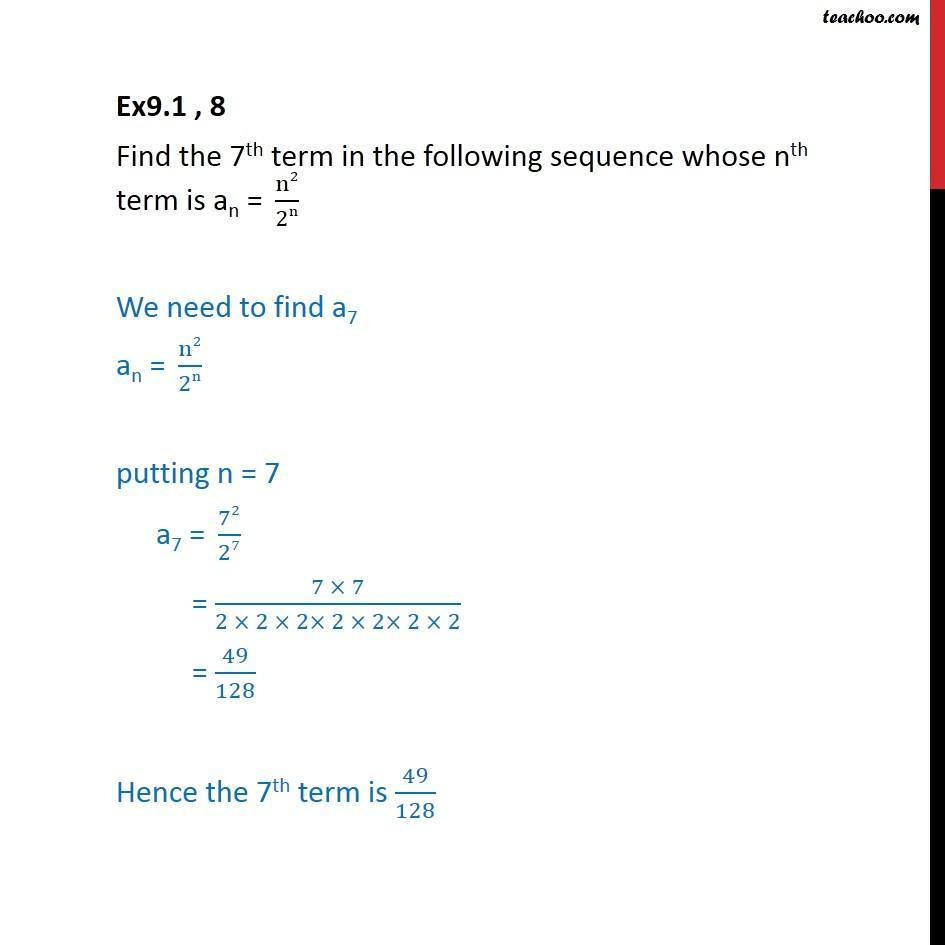Ex 8.1

Chapter 8 Class 11 Sequences and Series
Serial order wiseLearn in your speed, with individual attention - Teachoo Maths 1-on-1 Class

### Transcript

Ex9.1 , 8 Find the 7th term in the following sequence whose nth term is an =" " n2/2n We need to find a7 an =" " n2/2n putting n = 7 a7 =" " 72/27 = (7 7)/(2 2 2 2 2 2 2) = 49/128 Hence the 7th term is 49/128